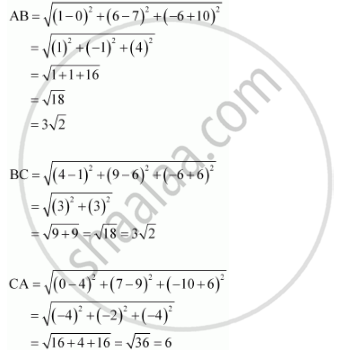CBSE (Arts) Class 11CBSE
Share

# Verify the Following: (0, 7, –10), (1, 6, –6) and (4, 9, –6) Are the Vertices of an Isosceles Triangle. - CBSE (Arts) Class 11 - Mathematics

ConceptThree - Dimensional Geometry Distance Between Two Points

#### Question

Verify the following: (0, 7, –10), (1, 6, –6) and (4, 9, –6) are the vertices of an isosceles triangle.

#### Solution

Let points (0, 7, –10), (1, 6, –6), and (4, 9, –6) be denoted by A, B, and C respectively.Here, AB = BC ≠ CA

Thus, the given points are the vertices of an isosceles triangle.

Is there an error in this question or solution?

#### APPEARS IN

NCERT Solution for Mathematics Textbook for Class 11 (2018 to Current)
Chapter 12: Introduction to Three Dimensional Geometry
Q: 3.1 | Page no. 273

#### Video TutorialsVIEW ALL 

Solution Verify the Following: (0, 7, –10), (1, 6, –6) and (4, 9, –6) Are the Vertices of an Isosceles Triangle. Concept: Three - Dimensional Geometry - Distance Between Two Points.
S